# AP Calculus AB Practice Test 47

### Test Information10 questions20 minutes

Calculator Disallowed

1. If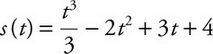for t≥ 0 is the position of a moving particle on a straight line, at which of the following intervals of tis the speed of the particle decreasing?

2.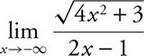3.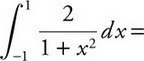4. If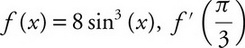is

5. Given the function f(x) = x3- 3x2+ 3x- 1, which of the following statements is true?

6. If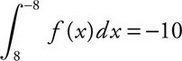and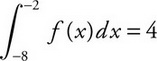, what is the value of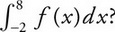7. What is the slope of the tangent line to the curve 2x2y- 3xy2- 2 = 0 at the point (2, 1)?

8. If fis a piecewise function defined as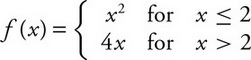, which of the following statements is/are true?

I.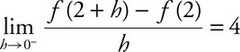II.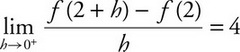III. f′(2) = 4

9. Air is pumped into a spherical balloon at the rate of 10 cubic centimeters per second. How fast is the diameter, in centimeters per second, increasing when the radius is 5 cm? (The volume of a sphere is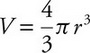.)

10.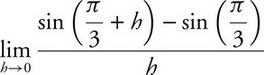is